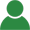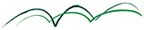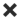ErrorHome Courses Course Calendar Teaching Team About Us Resources Contact
Course Calendar

# Factor Modeling for Investment Management

## Day One

### Background

• Overview factor research and applications: from the CAPM to "Smart Beta"
• Factor models: fundamental, macroeconomic, statistical, and hybrid
• Commercial versus custom factor models

### Statistical Foundations

• Linear regression models: assumptions, estimation, analytics (R^2, t-stats, F-test, DW)
• Cross-section versus time-series regressions in finance
• Overview advanced regression techniques: non-linear variables, dummy variables, non-linear estimation techniques, time-variable regression parameters

Workshop: Style analysis of a hedge fund

• Big Data & Data Mining: introduction to LASSO
• Limits to quantitative analysis: system complexity, data issues, stability, out-of-sample performance

Workshop: Identifying hedge fund performance factors

### Statistical Factor Models

• Understanding principal component analysis (PCA)

Workshops: Inferring the factor structure from single stocks, modeling yield curve dynamics, and identifying extreme scenarios for stress testing purposes

• Beyond PCA: Introduction to independent component analysis (ICA)

## Day Two

### Fundamental Factor Modeling

• Asset pricing and fundamental factors, factor-mimicking portfolios

Workshops: Building a fundamental factor model for an equity portfolio, modeling the momentum factor

### Macroeconomic Factor Modeling

• Real and monetary macroeconomic factors and transmission mechanisms

Workshop: Building a macroeconomic model for a multi-asset class portfolio, extracting factors from macroeconomic data

### Applications of Factor Models

• Performance Analysis
• Return contributions from factors
• True alphas & hidden factor exposures